# Studio Les 2 Alpes - Page 2

Viewing mode
224 results
•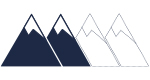• Slopes access :
• 50 -300m to the slopes
• Rooms :
• Studio
• Studio + cabin(s)
• Total surface (sq.m) :
• 33 sq.m
•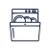•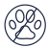•• Slopes access :
• 50 -300m to the slopes
• Rooms :
• Studio
• Studio + cabin(s)
• Total surface (sq.m) :
• 19 sq.m
•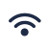•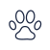•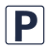••• Slopes access :
• ski-in (-50 m)
• Rooms :
• Studio
• Studio + cabin(s)
• Total surface (sq.m) :
• 30.87 sq.m
••••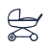•• Slopes access :
• 50 -300m to the slopes
• Rooms :
• Studio
• Studio + cabin(s)
• Total surface (sq.m) :
• 19 sq.m
•••••• Slopes access :
• 50 -300m to the slopes
• Rooms :
• Studio
• Total surface (sq.m) :
• 24 sq.m
•••••• Slopes access :
• 50 -300m to the slopes
• Rooms :
• Studio
• Studio + cabin(s)
• Total surface (sq.m) :
• 24 sq.m
••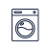•••• Slopes access :
• 50 -300m to the slopes
• Rooms :
• Studio
• Studio + cabin(s)
• Total surface (sq.m) :
• 19 sq.m
••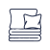•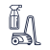•••• Slopes access :
• ski-in (-50 m)
• Rooms :
• Studio
• Studio + cabin(s)
• Total surface (sq.m) :
• 18,51 sq.m
••••• Slopes access :
• 50 -300m to the slopes
• Rooms :
• Studio
• Total surface (sq.m) :
• 34 sq.m
••••••• Slopes access :
• 50 -300m to the slopes
• Rooms :
• Studio
• Studio + cabin(s)
• Total surface (sq.m) :
• 21 sq.m
•••• Slopes access :
• ski-in (-50 m)
• Rooms :
• Studio
• Studio + cabin(s)
• Total surface (sq.m) :
• 30,49 sq.m
••••••• Slopes access :
• 50 -300m to the slopes
• Rooms :
• Studio
• Studio + cabin(s)
• Total surface (sq.m) :
• 25 sq.m
••••••• Slopes access :
• 50 -300m to the slopes
• Rooms :
• Studio
• Studio + cabin(s)
• Total surface (sq.m) :
• 24.14 sq.m
•••••• Slopes access :
• 50 -300m to the slopes
• Rooms :
• Studio
• Total surface (sq.m) :
• 18 sq.m
••••••• Slopes access :
• 50 -300m to the slopes
• Rooms :
• Studio
• Studio + cabin(s)
• Total surface (sq.m) :
• 34 sq.m
••••••• Slopes access :
• ski-in (-50 m)
• Rooms :
• Studio
• Studio + cabin(s)
• Total surface (sq.m) :
• 26.46 sq.m
•••••• Slopes access :
• ski-in (-50 m)
• Rooms :
• Studio
• Studio + cabin(s)
• Total surface (sq.m) :
• 24.75 sq.m
••••• Slopes access :
• 50 -300m to the slopes
• Rooms :
• Studio
• Total surface (sq.m) :
• 30 sq.m
••••• Slopes access :
• 50 -300m to the slopes
• Rooms :
• Studio
• Studio + cabin(s)
• Total surface (sq.m) :
• 19,10 sq.m
••••• Slopes access :
• 50 -300m to the slopes
• Rooms :
• Studio
• Studio + cabin(s)
• Total surface (sq.m) :
• 24.29 sq.m
••••